Courses

# Design Of Helical Springs For Variable Load (Part - 2) Mechanical Engineering Notes | EduRev

## Mechanical Engineering : Design Of Helical Springs For Variable Load (Part - 2) Mechanical Engineering Notes | EduRev

The document Design Of Helical Springs For Variable Load (Part - 2) Mechanical Engineering Notes | EduRev is a part of the Mechanical Engineering Course Machine Design.
All you need of Mechanical Engineering at this link: Mechanical Engineering

Extension springs

Part of an extension spring with a hook is shown in Fig.7.2.7. The nomenclature for the extension spring is given below.

Body length, LB : d ( N + 1 ) B

Free length, L : LB + 2 hook diameter.

B here, N stands for the number of active coils. By putting the hook certain amount of stress concentration comes in the bent zone of the hook and these are substantially weaker zones than the other part of the spring. One should take up steps so that stress concentration in this region is reduced. For the reduction of stress concentration at the hook some of the modifications of spring are shown in Fig 7.2.8.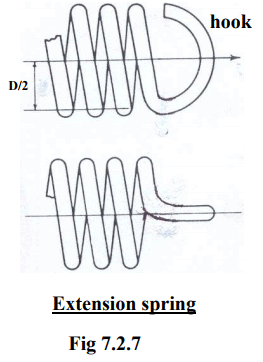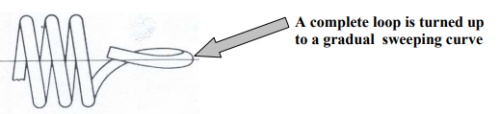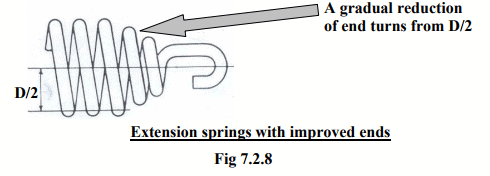Buckling of compression spring

Buckling is an instability that is normally shown up when a long bar or a column is applied with compressive type of load. Similar situation arise if a spring is too slender and long then it sways sideways and the failure is known as buckling failure. Buckling takes place for a compressive type of springs. Hence, the steps to be followed in design to avoid buckling is given below.

Free length (L) should be less than 4 times the coil diameter (D) to avoid buckling for most situations. For slender springs central guide rod is necessary.

A guideline for free length (L) of a spring to avoid buckling is as follows,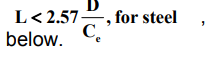(7.2.8)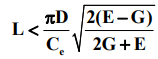Where, Ce is the end condition and its values are given

Ce       end condition
2.0      fixed and free end
1.0       hinged at both ends
0.707   hinged and fixed end
0.5       fixed at both ends

If the spring is placed between two rigid plates, then end condition may be taken as 0.5. If after calculation it is found that the spring is likely to buckle then one has to use a guide rod passing through the center of the spring axis along which the compression action of the spring takes place.

Spring surge (critical frequency)

If a load F act on a spring there is a downward movement of the spring and due to this movement a wave travels along the spring in downward direction and a to and fro motion continues. This phenomenon can also be observed in closed water body where a disturbance moves toward the wall and then again returns back to the starting of the disturbance. This particular situation is called surge of spring. If the frequency of surging becomes equal to the natural frequency of the spring the resonant frequency will occur which may cause failure of the spring. Hence, one has to calculate natural frequency, known as the fundamental frequency of the spring and use a judgment to specify the operational frequency of the spring.

The fundamental frequency can be obtained from the relationship given below.

Fundamental frequency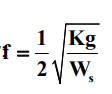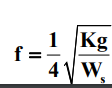Both ends within flat plates               (7.2.9)

One end free and other end on flat plate.                                                                     (7.2.10)

Where, K : Spring rate
WS : Spring

weight = 2.47 (7.2.11) γd2 DN                                                                                                (7.2.11)

and d is the wire diameter, D is the coil diameter, N is the number of active coils and γ is the specific weight of spring material.

The operational frequency of the spring should be at least 15-20 times less than its fundamental frequency. This will ensure that the spring surge will not occur and even other higher modes of frequency can also be taken care of.

A problem on spring design

A helical spring is acted upon by a varying load of 300 N to 900 N respectively as shown in the figure. The spring deflection will be around 15 mm and outside diameter of the spring should be within 48-50 mm.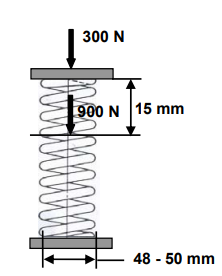Solution

To design the spring for the given data, the most important parameter is the spring index. The spring index decides the dimension of the spring with respect to chosen wire diameter. Normally the spring index varies over a wide range from 3-12. For higher value of the spring index the curvature effect will be less, but relatively size of the spring and stress in the spring wire will increase. However, the effects will be some what opposite if the value of spring index is lower. Hence, it is better to start the iteration process with the spring index of 6-7.

Let us start the problem with spring index, C=6 and wire diameter, d=7 mm.

The above choice gives us a coil mean diameter, D =42 mm. Thereby, the outside diameter of the coil is 49 mm, which is within the given limit. Computation of stresses:

The mean load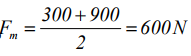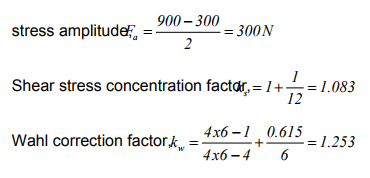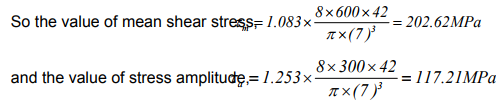Estimation of material properties:

As no specific use of the spring is mentioned in the problem, let us take Chrome Vanadium as the spring material. This alloy spring steel is used for high stress conditions and at high temperatures, it is also good for fatigue resistance and long endurance for shock and impact loads.

Ultimate strength of the material σut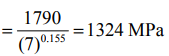From the relationship of σult to τy (yield point) and endurance limit,  τe we find that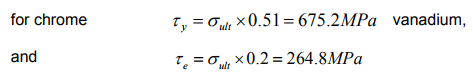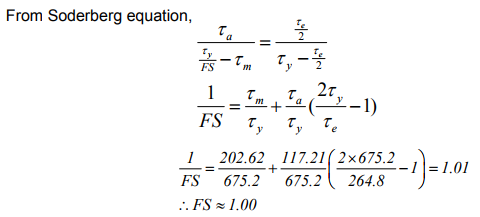Factor of safety, FS=1.0 implies that the design do not consider any unforeseen effect that may cause extra stresses in the spring. Normally in design of springs it is better to consider a factor of safety which should be in the vicinity of 1.3-1.5.

In order to increase the value of FS, in the next iteration, natural choice for the spring index, C is 5 and d = 8 mm. Because C=7 and d = 6 mm will lead to more stress on the wire and the value of FS will not improve. With C=5 and d=8 mm and following the similar procedure as in previous iteration we have,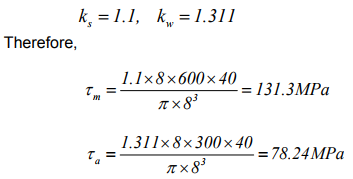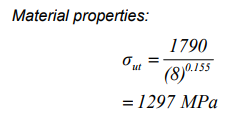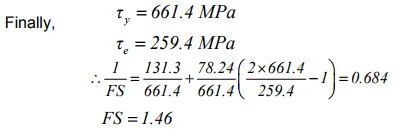The factor of safety obtained is acceptable. Therefore the value of spring index is 5 and corresponding wire diameter is 8mm.

Hence, mean spring diameter, D=40 mm.

Outer diameter of spring, Do=40+8=48 mm, This value is within the prescribed limit.

Inner diameter of spring, . D= 32mm

Spring rate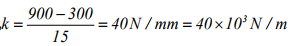Once the value of stiffness is known, then the value of number of active turns, N of the spring is,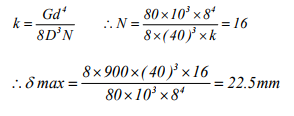In the above equation, G = 80000 MPa.

Spring Nomenclature:

Let us select the type of spring as squared and ground ends. For this type of spring the value of free length is,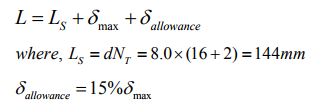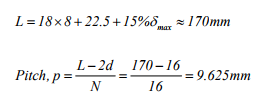Check for buckling:

We know that for steel,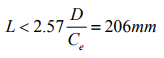Here, for the given spring seat configuration, Ce = 0.5

The free length of the spring, 170 mm is less than the critical length for buckling, 206mm. Therefore the design is safe.

Check for critical frequency:

In order to find the critical frequency of the spring, the weight of the spring is to be first computed,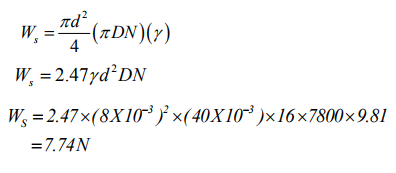Therefore,

The fundamental frequency of the spring (for both ends within flat plates),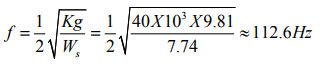Safe frequency for design should be at least 20 times less than the fundamental frequency to take care of more number of harmonics. Therefore, the spring frequency for should be around 6 Hz.

Offer running on EduRev: Apply code STAYHOME200 to get INR 200 off on our premium plan EduRev Infinity!

## Machine Design

56 videos|71 docs|94 tests

,

,

,

,

,

,

,

,

,

,

,

,

,

,

,

,

,

,

,

,

,

;